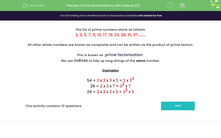# Practise Prime Factorisations Using Indices

In this worksheet, students will select the correct prime factorisation for the given numbers.Key stage:  KS 3

Curriculum topic:   Number

Curriculum subtopic:   Use Concepts and Vocabulary for All Numbers

Popular topics:   Prime Numbers worksheets, Factors worksheets

Difficulty level:#### Worksheet Overview

The list of prime numbers starts as follows:

2, 3, 5, 7, 11, 13, 17, 19, 23, 29, 31, 37........

In this activity, we will write numbers as the products of prime factors.

This is known as prime factorisation.

Method

All whole numbers, other than primes and the number 1, can be written as the product of their prime factors.

We find the prime factors of a number by successively dividing the number by its factor pairs.

Here is an example of a factor tree for the number 36, so that you can picture what they look like..36 has been split into its factor pair of 4 and 9.

4 has been split into its factor pair of 2 and 2. Both are prime numbers, so they have been circled.

9 has been split into its factor pair of 3 and 3. Both are prime numbers, so they have been circled.

So, the prime factors of 36 are 2, 2, 3 and 3.

36 as a product of its prime factors is: 3 x 3 x 2 x 2

We can tidy this up using indices to make 3² x 2²

Here are a few examples of numbers that have been broken down by prime factorisation:

54 = 2 x 3 x 3 x 3 = 2 x 33

28 x 2 x 22 x 7

24 2 x 2 x 2 x 3 23 x 3

Let's have a go at some questions now.

You can look back to this explanation at any time during the activity by clicking on the red button at the side of the screen.

### What is EdPlace?

We're your National Curriculum aligned online education content provider helping each child succeed in English, maths and science from year 1 to GCSE. With an EdPlace account you’ll be able to track and measure progress, helping each child achieve their best. We build confidence and attainment by personalising each child’s learning at a level that suits them.

Get started